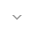# Camera

`public class Camera`
`extends Object`

 java.lang.Object ↳ android.graphics.Camera

## Summary

### Public constructors

` Camera() `

### Public methods

` void` ` applyToCanvas(Canvas canvas) `

` float` ` dotWithNormal(float dx, float dy, float dz) `
` float` ` getLocationX() `

` float` ` getLocationY() `

` float` ` getLocationZ() `

` void` ` getMatrix(Matrix matrix) `

` void` ` restore() `

` void` ` rotate(float x, float y, float z) `

` void` ` rotateX(float deg) `

` void` ` rotateY(float deg) `

` void` ` rotateZ(float deg) `

` void` ` save() `

` void` ` setLocation(float x, float y, float z) `

` void` ` translate(float x, float y, float z) `

### Protected methods

` void` ` finalize() `

### Inherited methodsFrom class ` java.lang.Object `

## Public constructors

### Camera

`Camera ()`

## Public methods

### applyToCanvas

`void applyToCanvas (Canvas canvas)`

Parameters
`canvas` `Canvas`: The Canvas to set the transform matrix onto

### dotWithNormal

```float dotWithNormal (float dx,
float dy,
float dz)```

Parameters
`dx` `float`
`dy` `float`
`dz` `float`
Returns
`float`

### getLocationX

`float getLocationX ()`

Returns
`float`

• `setLocation(float, float, float)`

### getLocationY

`float getLocationY ()`

Returns
`float`

• `setLocation(float, float, float)`

### getLocationZ

`float getLocationZ ()`

Returns
`float`

• `setLocation(float, float, float)`

### getMatrix

`void getMatrix (Matrix matrix)`

Parameters
`matrix` `Matrix`: The matrix to copy the current transforms into

### restore

`void restore ()`

• `restore()`

### rotate

```void rotate (float x,
float y,
float z)```

Parameters
`x` `float`: The angle of rotation around the X axis, in degrees
`y` `float`: The angle of rotation around the Y axis, in degrees
`z` `float`: The angle of rotation around the Z axis, in degrees

• `rotateX(float)`
• `rotateY(float)`
• `rotateZ(float)`

### rotateX

`void rotateX (float deg)`

Parameters
`deg` `float`: The angle of rotation around the X axis, in degrees

• `rotateY(float)`
• `rotateZ(float)`
• `rotate(float, float, float)`

### rotateY

`void rotateY (float deg)`

Parameters
`deg` `float`: The angle of rotation around the Y axis, in degrees

• `rotateX(float)`
• `rotateZ(float)`
• `rotate(float, float, float)`

### rotateZ

`void rotateZ (float deg)`

Parameters
`deg` `float`: The angle of rotation around the Z axis, in degrees

• `rotateX(float)`
• `rotateY(float)`
• `rotate(float, float, float)`

### save

`void save ()`

• `save()`

### setLocation

```void setLocation (float x,
float y,
float z)```

Parameters
`x` `float`: The x location of the camera
`y` `float`: The y location of the camera
`z` `float`: The z location of the camera

### translate

```void translate (float x,
float y,
float z)```

Parameters
`x` `float`: The distance to translate by on the X axis
`y` `float`: The distance to translate by on the Y axis
`z` `float`: The distance to translate by on the Z axis

## Protected methods

### finalize

`void finalize ()`

`Object``finalize`方法`Object`执行特殊操作; 它只是正常返回。 `Object`子类可能会覆盖此定义。

Java编程语言不保证哪个线程将为任何给定的对象调用`finalize`方法。 但是，保证调用finalize的线程在调用finalize时不会保留任何用户可见的同步锁。 如果finalize方法引发未捕获的异常，则忽略该异常，并终止该对象的终止。

`finalize`方法引发的任何异常 `finalize`导致终止此对象的终止，但会被忽略。

Throws
`Throwable`